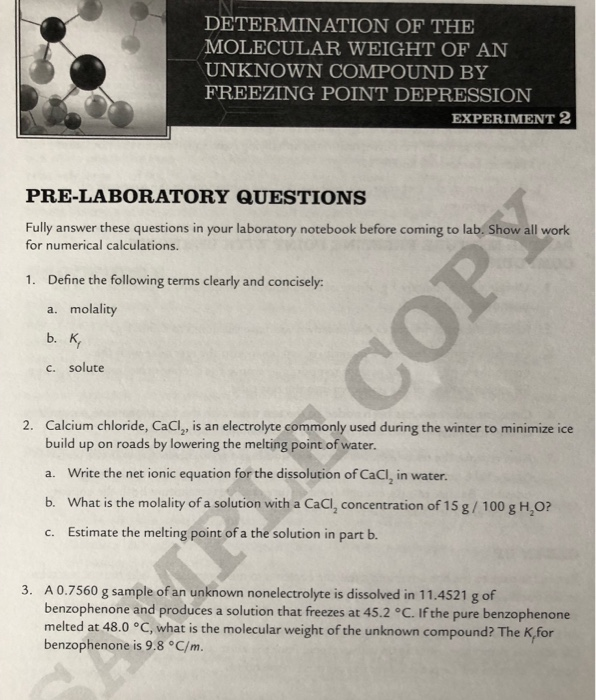Solved: DETERMINATION OF THE MOLECULAR WEIGHT OF AN UNKNOWN COMPOUND BY FREEZING POINT DEPRESSION EXPERIMENT 2 PRE-LABORATORY QUESTIONS Fully Answer These Questions In Your Laboratory Notebook Before

By |DETERMINATION OF THE MOLECULAR WEIGHT OF AN UNKNOWN COMPOUND BY FREEZING POINT DEPRESSION EXPERIMENT 2 PRE-LABORATORY QUESTIONS Fully answer these questions in your laboratory notebook before coming to lab. Show all work for numerical calculations. Define the following 1. clearly and concisely: terms molality a. b. K c. solute Calcium chloride, CaCl, is build up on roads by lowering the melting point of water. 2. electrolyte commonly used during the winter to minimize ice an Write the net ionic equation for the dissolution of CaCl, in water. a. What is the molality of a solution with a CaCl, concentration of 15 g/ 100 g H,O? b. Estimate the melting point of a the solution in part b. C. A 0.7560 g sample of an unknown nonelectrolyte is dissolved in 11.4521 g of benzophenone and produces a solution that freezes at 45.2 °C. If the pure benzophenone melted at 48.0 °C, what is the molecular weight of the unknown compound? The K,for benzophenone is 9.8 °C/m. 3.